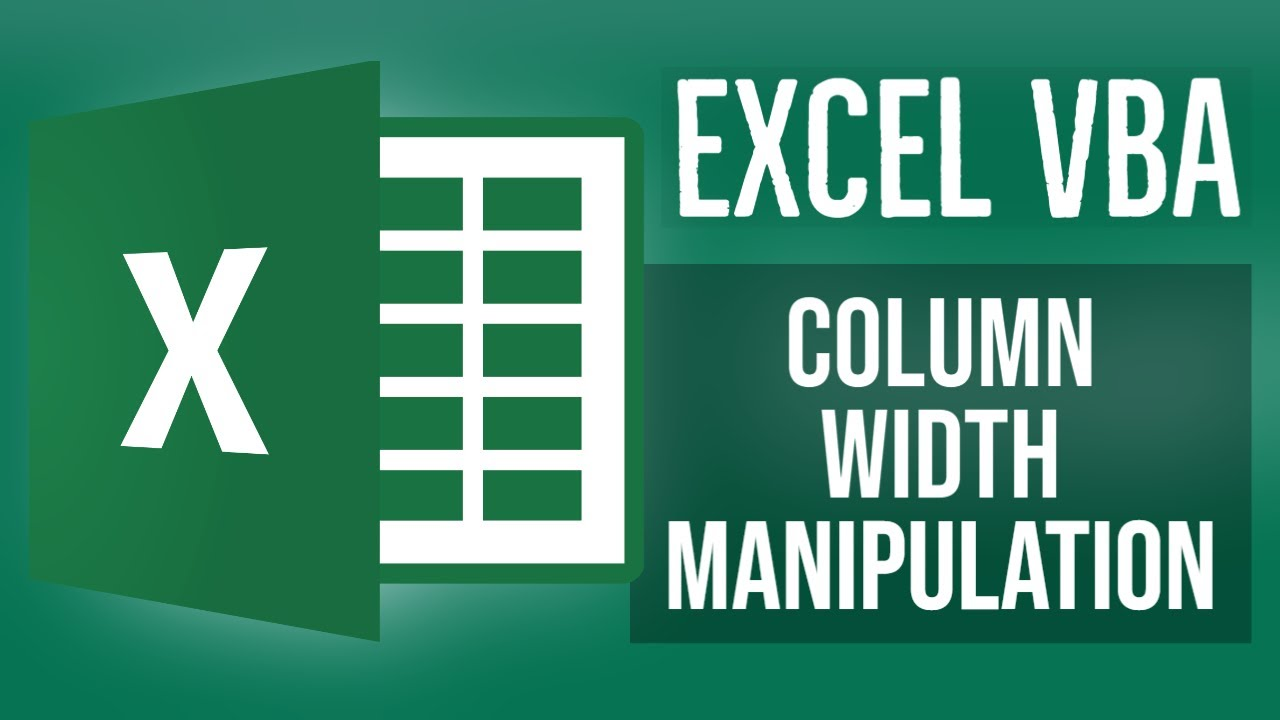# Excel VBA Tutorial for Beginners 20 - Column Width Manipulation in Excel VBAIn this MS Excel VBA video, we are going to see how can we use the VB code to manipulate the Column Width of Cells in MS Excel. So we are going to use two methods and explore them in detail, along with this we aare also going to see that what are the possible values for the width of columns

In this MS Excel VBA video, we are going to see how can we use the VB code to manipulate the Column Width of Cells in MS Excel. So we are going to use two methods and explore them in detail, along with this we aare also going to see that what are the possible values for the width of columns

Welcome to the The Beginner's Guide course to Excel VBA (Visual Basic for Applications). This course enables you to Learn MS Excel VBA in simple and easy steps. In this Microsoft Excel Basics Tutorial series we will start from the basics and gradually move towards the Expert level in Microsoft Excel VBA. This MS Excel VBA course provides the Beginners to Intermediate Excel VBA Skills, Tips, and Tricks. In this course we will learn how to Enter and edit Excel data, Format numbers, fonts and alignment, Make simple pivot tables and charts, Create simple Excel formulas, How to Use Excel Functions IF and VLOOKUP. Learn common Excel functions used in any Office, How to Create dynamic reports, Build Excel formulas to analyze date, text fields, values and arrays and much more advanced stuff.

In this video we will see the Overview of formulas in Excel. We will see Basic Excel formulas & functions with examples .

## Excel VBA Tutorial for Beginners 17- Delete Cells using VBA in MS Excel

In this MS Excel VBA video, we are going to see that how can we delete cells in excel using the VB code in the VBA editor and the Overview of formulas in Excel. We will see Basic Excel formulas & functions with examples .

## Excel VBA Tutorial for Beginners 16 - Clear Cells in Excel using VBA

In thi MS Excel VBA video, we are going to look at how can we use the VB code to clear up cells in MS Excel. The clear cells feature of MS Excel is used for the purpose. Using this feature we can easily clear cell contents, cell formatting, cell comments and hyperlinks as well

## Excel VBA Tutorial for Beginners 10 - Background Colors in Excel VBA

In this Excel VBA video, we are going to see the usage of With Block in Excel VBA. Using with block, we can reuse and rewrite multiple code lines. Also we are going to look at the interior property in brief as well, which allows us to set background colors and background gradient as well

## Excel VBA Tutorial for Beginners 11 - Excel VBA PasteSpecial Method

In this Excel VBA video, we are going to look at the usage of the Paste Special function in Excel using the VB code. Paste Special function helps us to use the paste function in different formats such as Pasting Cell Width, Pasting Text only, Pasting Cell Formats.

## Excel VBA Tutorial for Beginners 19 - VBA Insert Cells in MS Excel

In this MS Excel VBA video, we are going to see the usage of the Insert Cells feature of MS Excel. Using this feature we can insert cells at some specified locations easily. In this video we will see the Overview of formulas in Excel.